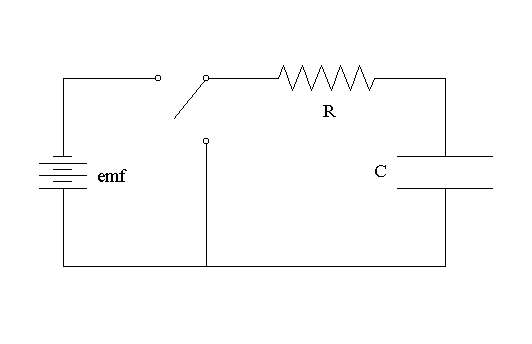# 4. Circuits (Resistors and Capacitors)

Now that electric fields, voltage, and current have been explained, we can introduce circuits. Circuits are networks that connect various electrical elements such as voltage sources (i.e. batteries), resistors, and capacitors. Below are listed the various parts of a circuit which may be crucial for understanding solar technology.

• A voltage source, or source of emf (electromotive force),is some device that creates a potential difference between two points, thus generating a current through a circuit. A common example is the battery, which converts chemical energy (involved in chemical reactions within the battery) into electrical energy.
• Capacitors are places in the circuit where at least two conductor surfaces are separated by some insulator. If a voltage source is present in the circuit, a capacitor will store charge, gradually charge up, and eventually prevent current flow upon full charge. A common example is two parallel metal plates, where opposite charges accumulate on each plate.
• Resistors are circuit elements which can be added to limit current flow, following the equationwhere V is voltage, I is current (measured in Amps, A) and R is resistance (measured in Ohms, Ω). In other words, the lower the resistance and current, the lower the voltage in the circuit. Resistance in a circuit can also represent the natural resistance of the wires or other components themselves.Above: A circuit diagram, including a battery (emf), capacitor (C), a resistor (R), and a switch that redirects current and therefore switches off the source of voltage.

Source: onlinephys.com. 4 March 2012.

<onlinephys.com/electricoscillator.html>